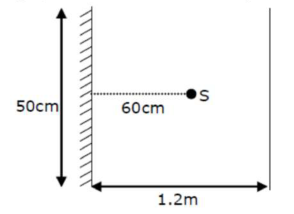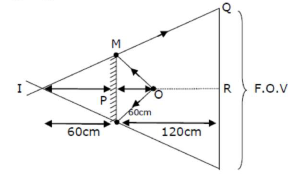# A point source of light S, placed at a distance 60 cm

Question:

A point source of light $S$, placed at a distance $60 \mathrm{~cm}$ infront of the centre of plane mirror of width $50 \mathrm{~cm}$, hangs vertically on a wall. A man walks infront of the mirror along a line parallel to the mirror at a distance $1.2 \mathrm{~m}$ from it (see in the figure). The distance between the extreme points where he can see the image of the light source in the mirror is $\mathrm{cm}$Solution:

(150)

from similar triangle IMP and IQR

$\frac{Q R}{25}=\frac{180}{60} \Rightarrow Q R=7$F.O.V. $=2 \times 75 \Rightarrow 150 \mathrm{~cm}$